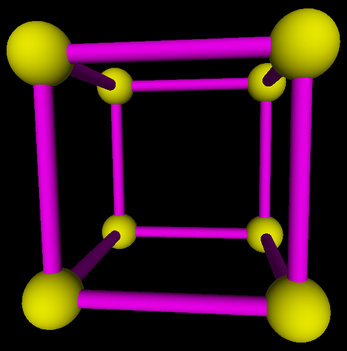## CS 424: Computer Graphics, Fall 2017 Lab 4: Introducing OpenGL 1.1

This is our first lab on 3D graphics. You will be working with OpenGL 1.1, drawing and transforming simple shapes. You can work either in Java or in C. If you use Java, you should work on a copy of the file Lab4.java. If you use C, should get the file lab4.c instead. You can find a copy of each file in /classes/cs424

We have not yet covered a lot of things that are necessary for realistic-looking 3D scenes. To avoid disappointing you with the look of the scenes in this lab, the starter files do some setup in their initialization subroutines that you are not expected to understand. In particular, they set up a perspective projection and turn on some basic lighting effects.

To program in Java, you will need to set up Eclipse to use the JOGL API. See Subsection 3.6.2 for instructions on doing that. The basic idea is to make a "User Library" containing the JOGL files. Then you can start a new project and add the JOGL user library to the project. There are copies of the JOGL .jar files and the native libraries for Linux in /classes/cs424/JOGL-support. (Note: There is no need to make copies of these files, if you are using them on Linux. Your User Library can can refer directly to the copies in /classes/cs424/JOGL-support.) You can also get copies of the .jar files and the native libraries for Linux, Windows, or Mac from http://math.hws.edu/eck/cs424/jogl_2_3_support/. Be sure to read all the instructions in the textbook and follow them carefully!

For C programming, there is no special setup. The OpenGL libraries for C are installed in Linux on the lab computers. However, we have not installed an IDE for C programming. You can edit your C programs in a text editor such as xed or Komodo Edit, and you can compile and run them on the command line. For this lab, you can use this compilation command:

```      gcc -o lab4 lab4.c -lGL -lglut
```

The program can then be run with the command

```     ./lab4
```

If you need to print out debugging messages in C, use the standard IO function, printf, which works in the same way Java's System.out.printf. That is, you can use it to print out a string (usually with a "\n" at the end for a carriage return), and you can use it with a format string to print out the values of variables. For example,

```     printf("n is %d, x is %1.5f\n", n, x);
```

Turning in your work: Your completed program must be submitted by the beginning of the lab period next Thursday. You can just copy the completed lab4.c or Lab4.java directly into your homework folder in /classes/cs424/homework. (Or put it inside a folder named lab4 if you want.)

### A Note About Anaglyph Stereo

To add a little interest to the lab, the program that you will be working on implements an "anaglyph stereo" view of the scene. An anaglyph stereo image is meant to be viewed through red/cyan 3D glasses. (I will give you a pair in lab.) On the screen, the view from the left eye is drawn using only shades of red, and the view from the right eye uses only shades of green and blue. In the program, anaglyph mode is turned on and off by pressing the space bar. Since color anaglyph didn't work very well, the program turns color off when in anaglyph mode. Here's how the program will draw the "cage" that you make at the end of the lab, in anaglyph mode: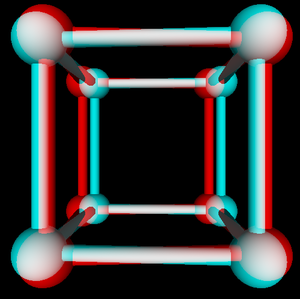You do not have to do any work for this part of the lab. I will explain how it works in class. However, here's the basic idea:

1. Move the viewer to the position of the left eye, and aim the view towards the object;
2. Draw the scene as usual, but writing only to the red color channel;
3. Clear the depth buffer (but NOT the color buffer!);
4. Move the viewer to the position of the right eye, and aim the view towards the object;
5. Draw the scene as usual, writing only to the green and blue color channels;
6. Go back to drawing to all color channels.

(Do you see why step number 3 is essential?) Note that the transformations have to be applied first, before any modeling transformations.

The only new OpenGL feature that is needed to implement all this is the ability to control which color channels are being written. The function

```glColorMask( r, g, b, a );
```

can do that. It is not mentioned in the textbook. The parameters to glColorMask are boolean values. (For Java, true and false are used; for C, 1 and 0 are used to represent the boolean values.) The parameters r, g, b and a control writing to the red, green, blue, and alpha color channels, respectively. A true value means that it is possible to write to the channel; a false value blocks writing to the channel. In the initial state, of course, all the color channels are enabled. To restore the initial state, call glColorMask with all parameters set to true.

### Draw a Shape

For this lab, you will be drawing six objects using OpenGL. When you run the completed program, hitting one of the number keys 1 through 6 will select the object that is displayed. The program already sets the value of a global variable, objectNumber, to tell you which object to draw. The user can rotate the object using the arrow, PageUp, PageDown, and Home keys. The display() subroutine is called to draw the object. That subroutine in turn calls draw(), and it is inside draw() where you should do the basic work. (The place is marked with a TODO.) You will also add a few new subroutines to the program.

Object number 1 is a simple 2D shape, which you should draw directly, using glBegin(), glEnd(), glVertex2f(), and glColor3f. You should use different colors for different vertices. Here is the shape, with the vertices labeled: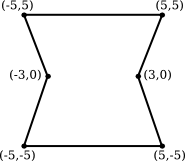It is possible to make this shape using a single primitive or type GL_TRIANGLE_FAN, or GL_TRIANGLE_STRIP, or GL_QUAD_STRIP.

### Draw a Wireframe Polyhedron

Object number 2 is a wireframe polyhedron. That is, you will just draw the edges of the polyhedron. The particular polyhedron is called a stellated dodecahedron. It has 60 triangular faces. The data for the polyhedron is already in the program, in two two-dimensional arrays named dodecVertices and dodecTriangles.

The first array, dodecVertices, is a 2D array of double that contains the (x,y,z) coordinates of the vertices of the polyhedron. For example, vertex number 13 has coordinates

```         (dodecVertices, dodecVertices,  dodecVertices)
```

You can use these coordinates in the function glVertex3d(x,y,z), or you can use the entire array in glVertex3dv(array). (In Java, remember, the functions are gl2.glVertex3d(x,y,z) and gl2.glVertex3dv(array,0).)

The second array, dodecTriangles, is a 2D array of int. Each row of the array contains three integers, giving the vertex numbers for the vertices of one of the triangular faces of the polyhedron. That is, the integers in dodecTriangles are indices into the vertex array. For example, dodecTriangles is the vertex number for the second vertex of face number 7.

Your job is to draw the outline of each of the 60 triangular faces. You can do that by drawing each face separately as a primitive of type GL_LINE_LOOP for each face. Using the two arrays can easily get confusing. When processing face number i, I suggest that you give names to the vertex numbers for that face. For example,

```int b = dodecTriangles[i];  // Index in dodecVertices for vertex 1 of face i.
```

(If you still find this confusing, you can look ahead at the discussion of "Indexed Face Sets" in Subsection 3.4.1. But remember that here you are drawing the edges, not the faces, of the polyhedron.)

Important Note: A wireframe should be drawn with lighting effects turned off! To implement that, you should use the command

```glDisable(GL_LIGHTING);  // or gl2.glDisable(GL2.GL_LIGHTING) in Java
```

before you draw the polyhedron, and

```glEnable(GL_LIGHTING);  // or gl2.glEnable(GL2.GL_LIGHTING) in Java
```

after you draw the polyhedron.

(One more note: The initialization subroutine calls glLineWidth(3), to make lines 3 pixels wide. The stellated dodecahedron is the only shape that uses lines, so this setting only applies to that shape. The wider lines make the shapes more distinct.)

### Use GLUT (and Transforms)

Object number 3 is a very simple example of using GLUT and transforms. This is just a warm-up for the remaining objects. The third object is a solid, 3D object that consists of a green cone sitting on top of a brown cylinder, meant to look a little like a tree. Here are to pictures of the tree in slightly different orientations so that you can see that cone actually has no bottom (which is unfortunate, but we will put up with it):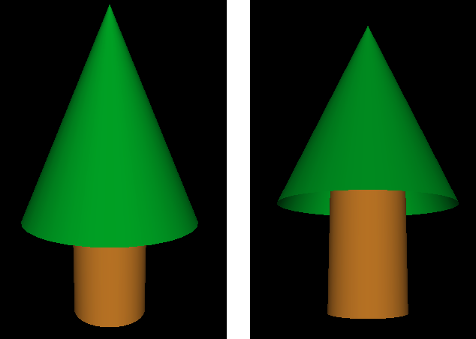The cone and the cylinder can be drawn using the GLUT library:

```glutSolidCylinder( radius, height, 32, 8 );

glutSolidCone( radius, height, 32, 8 );
```

In Java, the method names will be glut.glutSolidCylinder and glut.glutSolidCone. The first two parameters specify the size of the object. The last two are "slices" and "stacks" and determine how smooth the curved surfaces will be; the values 32 and 8 are fine for this lab.

For this example, my cone has radius 3.5 and height 8, my cylinder has radius 1.5 and height 5, and the cylinder sticks 1 unit into the interior of the cone..

Both the cone and the cylinder are drawn with the center of their bases at (0,0,0) and with their axes lying along the positive z-axis (that is, pointing directly out of the screen, assuming no transformation has been applied). So, if you just use the above commands, the cone will be in the same locations! Obviously, you will need to apply a transformation. In the end, the complete object should have its center at (0,0,0) for proper display. It will still be pointing directly at you, so you will have to rotate it to get a better view.

Don't forget to use glPushMatrix() and glPopMatrix() to limit the effect of any transformation that you apply!

### Build a Cage

Objects number 4, 5, and 6 are stages in building a "cage" out of cylinders and spheres. The cage, object number 6, has eight spheres at the vertices of a cube. The centers are the points (-4,-4,-4), (-4,-4,4), (-4,4,-4), etc. There is a narrow cylinder lying along each edge of the cube. You should use different colors for the cylinders and for the spheres. Note that a sphere can be drawn using the GLUT function

```glutSolidSphere( radius, 32, 32 );
```

or glut.glutSolidShpere for Java. The center of the sphere is at (0,0,0). The first parameter is the radius. The other two parameters are, again, "slices" and "stacks"; you can use the value 32 for this lab.

Object number 4 is a "bar" consisting of a cylinder with a translated sphere at each end. The cylinder lies along the x-axis, and the centers of the spheres are at (-4,0,0) and (4,0,0):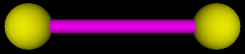You should write a subroutine to draw the bar. Note that for Java, you will need to pass gl2 as a parameter to the subroutine.

Object number 5 is square shape that can be made out of two translated copies of the bar, plus two additional cylinders. The square lies in the xy-plane, and the centers of the four spheres are at (-4,4,0), (4,4,0), (-4,-4,0), and (4,-4,0). (Do not make the square out of four bars — that would actually contain eight spheres, not four.) Again, you should write a subroutine to draw the square: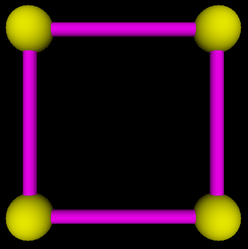Finally, object number 6 is the full cage, which can be made from two translated copies of the square, plus four additional cylinders. It should be centered at (0,0,0):